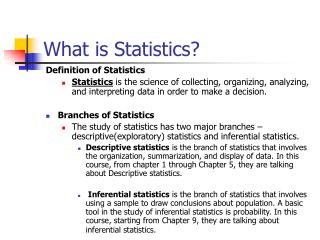DownloadDownload PresentationWhat is Statistics?

# What is Statistics?

Télécharger la présentation## What is Statistics?

- - - - - - - - - - - - - - - - - - - - - - - - - - - E N D - - - - - - - - - - - - - - - - - - - - - - - - - - -
##### Presentation Transcript

1. What is Statistics? Definition of Statistics • Statistics is the science of collecting, organizing, analyzing, and interpreting data in order to make a decision. • Branches of Statistics • The study of statistics has two major branches – descriptive(exploratory) statistics and inferential statistics. • Descriptive statistics is the branch of statistics that involves the organization, summarization, and display of data. In this course, from chapter 1 through Chapter 5, they are talking about Descriptive statistics. • Inferential statistics is the branch of statistics that involves using a sample to draw conclusions about population. A basic tool in the study of inferential statistics is probability. In this course, starting from Chapter 9, they are talking about inferential statistics.

2. Chapter 1 Picturing Distributions with Graphs

3. Chapter outline • Individuals and variables • Categorical variables: • Pie Charts and bar graphs • Quantitative variables: • Histograms • Interpreting histograms • Quantitative variables: Stemplots • Time plots

4. Examining Distributions- Introduction • Definitions: • Individuals: the objects described by a set of data • Variable: any characteristic of an individual

5. Examples • College student data: every currently enrolled student – date of birth, gender, major, GPA and so on • Employee data: every employee – age, gender, salary, job type

6. Variables • Categorical variable: categories, groups • Quantitative variable: numerical values • Distribution of a variable: what values it takes and how often it takes these values

7. Examples • College student data: every currently enrolled student – DOB, gender, major, GPA, and so on • Employee data: every employee – age, gender, salary, job type • We can see distributions easily using graphs. It is possible to see distributions using numbers which describe the data.

8. Example 1.1 (Page 5)

9. Exploratory data analysis describes the main feature of data. • 1. Examine each variable • 2. Study the relationships among the variables • 3. Start with graphs and add some numerical summeries.

10. Categorical variables--- bar graphs and pie charts • Distribution of categorical variables categories by relevant count or percent of individuals. • Graphs: bar graph, pie chart • Pie chart: figure 1.1 (P. 7)/ must include all categories • Bar graph: figure 1.2 (P. 8)/heightindividual’s weight [gaps between bars and order is not important.] • Note: It’s only for single variable now (for example: college major, tire model, final exam grade).

11. Quantitative variables: histograms • How to make histograms • Step 1. Choose the classes. Divide the range of the data into classes of equal width. • Step 2. Count the individuals in each class. • Step 3. Draw the histogram. • Example 1.3

12. Example 1.3 (P. 11)

13. Interpreting histograms • Interpretation: What do we see? Overall pattern and striking deviations. • Overall pattern Shape, center, spread: symmetric, skewed to the right/left, clustered. • striking deviations Outlier

14. Example 1.5 (P. 13)

15. Example 1.6 (P. 14)

16. Quantitative variables: stemplots • Another way to display a distribution of quantitative variables. • How to make stemplots • 1. Sort data in increasing order first • 2. Separate each observation into a stem consisting of all but the final digit, and a leaf, the final digit. • 3. Write the stems in a vertical column with the smallest at the top, and draw a vertical line at the right of this column • 4. Write each leaf in the row to the right of its stem, in increasing order out from the stem.

17. Quantitative variables: stemplots • Data: 80, 52, 86, 94, 76, 48, 92, 69, 79, 45 • Step 1. Sort data in increasing order first • Step 2. Decide stem • Step 3. Fill in leaves

18. Examples and Exercises • Example 1.7 (P. 16) using Table 1.1 (P. 10) • Example 1.8 (P. 16)

19. Tips • 1. Rounding • 2. Splitting stems

20. Quantitative variables: stemplots • For small data sets, it is quicker to make and presents more detailed information • You keep data values

21. Time plots • It is for variables which are measured at intervals over time. • Example 1. The cost of raw materials for a manufacturing process each month. • Example 2. The price of a stock at the end of each day.

22. Time plots • To display change over time, make a time plot. Plot each observation against the time at which it was measured • 1. Put time on the horizontal scale • 2. Put the variable on the vertical scale • 3. Connect the data points by lines • Special case: time series (for regularly measured variable) • You can see: 1 )seasonal variation, 2) trend

23. Example 1.9 (P.18)

24. Free tutoring The Math Assistance Complex (MAC) 122 Kell Hall • MAC website:(online tutoring available) www.gsu.edu/~wwwclc/mathlab.htm